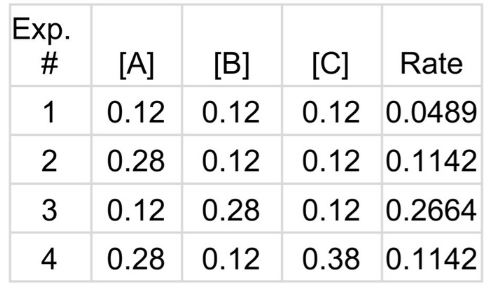# Problem: Given the initial concentrations in  Kinetics Data Table 1, predict what the rate would be if the initial concentrations were [A] = 0.062 M, [B] = 1.03 M, and [C] = 1.12 M.2 A + 3 B + C → 2 D + Ea. 2.026 M/sb. 0.126 M/sc. 2.086 M/sd. 1.863 M/se. 0.112 M/s

###### FREE Expert Solution
100% (369 ratings)
###### Problem Details

Given the initial concentrations in  Kinetics Data Table 1, predict what the rate would be if the initial concentrations were [A] = 0.062 M, [B] = 1.03 M, and [C] = 1.12 M.

2 A + 3 B + C → 2 D + Ea. 2.026 M/s

b. 0.126 M/s

c. 2.086 M/s

d. 1.863 M/s

e. 0.112 M/s

What scientific concept do you need to know in order to solve this problem?

Our tutors have indicated that to solve this problem you will need to apply the Rate Law concept. You can view video lessons to learn Rate Law. Or if you need more Rate Law practice, you can also practice Rate Law practice problems.

What is the difficulty of this problem?

Our tutors rated the difficulty ofGiven the initial concentrations in  Kinetics Data Table 1, ...as high difficulty.

How long does this problem take to solve?

Our expert Chemistry tutor, Jules took 9 minutes and 3 seconds to solve this problem. You can follow their steps in the video explanation above.

What professor is this problem relevant for?

Based on our data, we think this problem is relevant for Professor Sheng's class at FGCU.